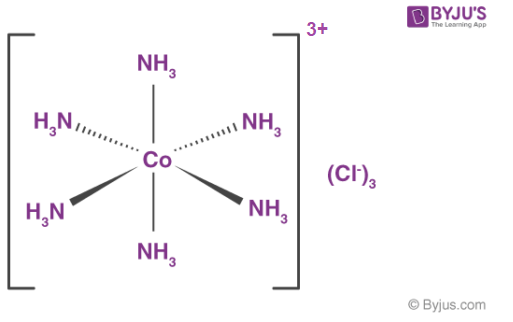# Coordination Number

The Coordination number of an atom in a given molecule or a crystal refers to the total number of atoms, ions, or molecules bonded to the atom in question. ‘Ligancy’ is another term used to refer to the coordination number of an atom.

The atoms, ions, or molecules that are bonded to the central atom (or molecule/ion) are called ligands. The ligancy of molecules is calculated differently when compared to calculating the coordination number of a central atom in a crystal.

## Coordination Number of a Central Atom

In the case of polyatomic ions and molecules, the coordination number corresponding to a given atom can be calculated by counting the total number of atoms it is bonded to, be it a single bond or a double/triple bond.

Considering the example of the polyatomic ion given by the formula [Cr(NH3)2Cl2Br2], the coordination number of the central cation (Cr3+) can be counted by the total number of atoms bonded to the chromium atom, which is found to be 6.In the example provided above, it can be observed that the coordination number of the central cobalt atom is 6 since it is bonded to 6 different nitrogen atoms.

For crystals, the bonds are not as clear in their solid state structures. In such cases, the value of the coordination number of the central atom equals the total number of neighbouring atoms to the atom in question.

The total number of neighbouring atoms to a specific atom in a crystal depends on the location of the atom in the crystal. Therefore, there are 2 different measures for ligancy in the case of crystals, namely the bulk coordination number and the surface coordination number.

In the case of coordination complexes, only the sigma bonds between the ligands and the central atom are counted in the calculation of the coordination number of the central atom. Pi bonds are disregarded in this calculation.

For example, in the compound tungsten hexacarbonyl, given by the chemical formula W(CO)6,  the coordination number of the central tungsten atom (denoted by the symbol W) is 6 despite the importance of pi bonding along with sigma bonding in such metal carbonyls.

## Geometry of Molecules Based on Coordination Number

There exist multiple possible geometric combinations for each value of the coordination number for the central atom. These possible geometric shapes are tabulated below.

 Coordination Number Geometric Structure 2 Linear 3 Trigonal planar, T-shaped, or trigonal pyramidal 4 Square planar or tetrahedral 5 Trigonal bipyramidal or square pyramid structures 6 Trigonal prism structure, hexagonal planar, or octahedral 7 Pentagonal bipyramidal, capped octahedron, or a capped trigonal prism structure. 8 Cubic, hexagonal bipyramidal, square antiprism, or dodecahedron 9 Three-face centered trigonal prism 10 A bicapped square antiprism structure 11 All faced capped trigonal prism structure 12 Cuboctahedron structure

## Frequently Asked Questions – FAQs

Q1

### What do you mean by coordination number?

Coordination number, also known as ligancy, is the number of atoms, ions, or molecules that a central atom or ion carries in a complex or coordination compound or in a crystal as its closest neighbours.

Q2

### What is a coordination reaction?

A coordination complex is the result of a Lewis acid-base reaction where, by coordinating covalent bonds, neutral molecules or anions (called ligands) bind to a central metal atom (or ion). They contain at least one pair of electrons to donate to a metal atom / ion. Ligands are Lewis bases.

Q3

### What is a unit cell?

The unit cell is defined as the smallest repeating unit having the full symmetry of the crystal structure. The geometry of the unit cell is defined as a parallelepiped, providing six lattice parameters taken as the lengths of the cell edges (a, b, c) and the angles between them (α, β, γ).

Q4

### What is square close packing?

In two-dimensional close packing, to achieve a two-dimensional pattern, rows of closed packed spheres are stacked. This stacking is achieved in two ways: Square close packing: In a close packing, the second row can be located precisely below the first row. Each sphere in such an arrangement is in contact with four other spheres.

Q5

### What is face Centred unit cell?

An arrangement of crystal atoms in which the atomic centres are disposed of in space in such a manner that one atom is positioned at each of the cube’s corners and one at each face’s centre. In a cubic, closest-packed structure, the face-centered cubic unit cell is the simplest repeated unit.

Test your knowledge on Coordination Number